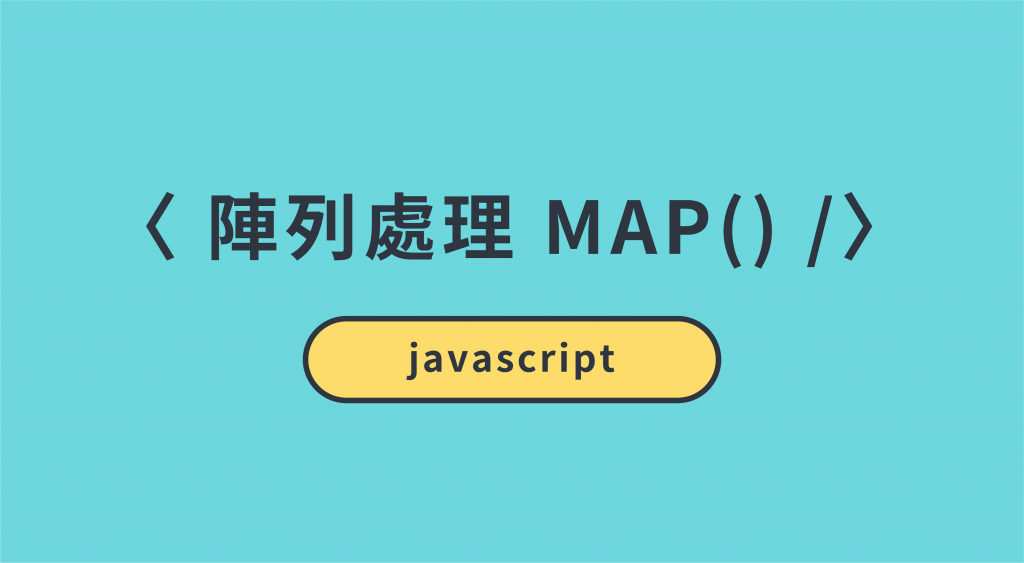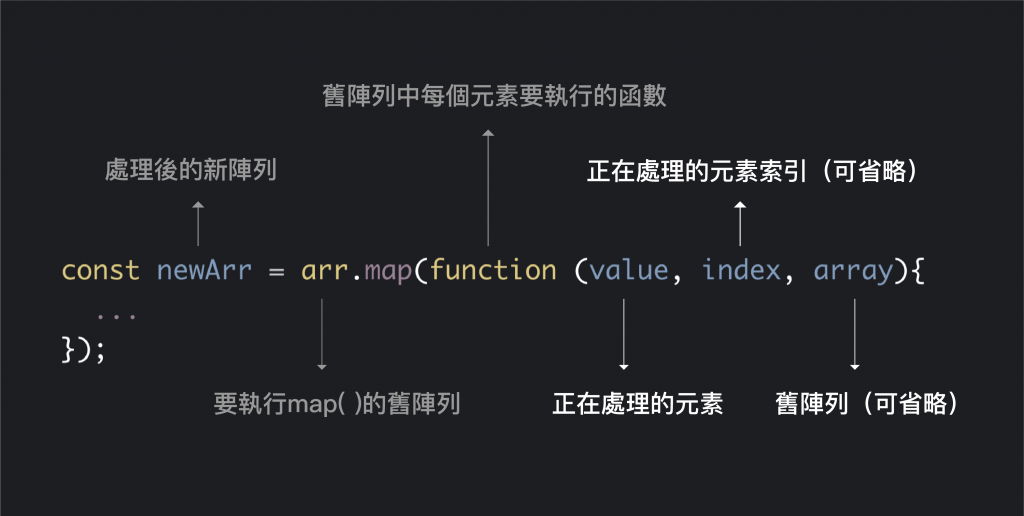#DAY 13
1
Modern Web

## Day13【ES6 小筆記】Array.map() - 處理陣列最佳選擇11.1 不要使用迭代器。更好的做法是使用 JavaScript 的高階函式，像是 `map()``reduce()`，替代如for-of 的迴圈語法。

### `map()` 特色

• 會透過`函式`內所回傳的值組合成一個新的陣列
• 並不會改變原陣列
• 回傳數量會等於原始陣列的長度
• 如果不回傳則是 `undefined`

#### 使用語法

``````const newArr = arr.map(function (value, index, array){
//...
});
``````### 範例

``````let A = [9000, 8500, 5500, 6500];
let B = A.map(function (value, index, array) {
return value*2;
});

console.log(A) // [9000, 8500, 5500, 6500] - 原陣列不會被修改
console.log(B) // [18000, 17000, 11000, 13000] - 發財摟 ^____^
``````

#### 使用箭頭函示的縮寫就會變這樣

``````let A = [9000, 8500, 5500, 6500];
let B = A.map(item => item*2);
console.log(B) // [18000, 17000, 11000, 13000] - 還是發財摟 ^____^
``````

### 【小補充】

`map()` 方法使用起來跟 `forEach()` 方法非常類似，最大的區別就是 `forEach()` 會修改原本的陣列，而`map()` 方法可以回傳一個新的陣列，並保有原本的舊陣列，另外 `forEach()` 並不會回傳任何東西，所以寫在 return 裡的東西不會有作用哦！

#### foreach() 範例

``````let A = [9000, 8500, 5500, 6500];
let B = A.map(function (value, index, array) {
return value*2; // forEach 沒有在 return 的，所以不會有作用
});
console.log(B); // undefined
``````

``````let A = [9000, 8500, 5500, 6500];
A.forEach(function (value, index, array) {
array[index] = value*2;
});
console.log(A); // [18000, 17000, 11000, 13000] - 發財摟 ^____^
``````

### 3 則留言

0forEach 沒有return

``````let B = A.map(function (value, index, array) {
``````

0

0